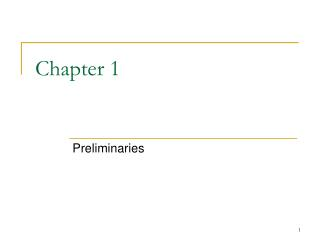# Chapter 1 - PowerPoint PPT PresentationDownload PresentationChapter 1

Chapter 1
Download Presentation## Chapter 1

- - - - - - - - - - - - - - - - - - - - - - - - - - - E N D - - - - - - - - - - - - - - - - - - - - - - - - - - -
##### Presentation Transcript

1. Chapter 1 Preliminaries

2. 1.3 Functions and Their Graphs

3. Function • y = f(x) • f represents function (a rule that tell us how to calculate the value of y from the variable x • x : independent variable (input of f ) • y :dependent variable (the correspoinding output value of f at x)

4. Definition Domain of the function The set of D of all possible input values Definition Range of the function The set of all values of f(x) as x varies throughout D

5. Natural Domain • When a function y = f(x)is defined and the domain is not stated explicitly, the domain is assumed to be the largest set of real x-values for the formula gives real y-values. • e.g. compare “y = x2” c.f. “y = x2, x≥0” • Domain may be open, closed, half open, finite, infinite.

6. Verify the domains and ranges of these functions

7. Graphs of functions • Graphs provide another way to visualise a function • In set notation, a graph is {(x,f(x)) | xD} • The graph of a function is a useful picture of its behaviour.

8. Example 2 Sketching a graph • Graph the function y = x2 over the interval [-2,2]

9. The vertical line test • Since a function must be single valued over its domain, no vertical line can intersect the graph of a function more than once. • If a is a point in the domain of a function f, the vertical line x=a can intersect the graph of f in a single point (a, f(a)).

10. Piecewise-defined functions • The absolute value function

11. Graphing piecewise-defined functions • Note: this is just one function with a domain covering all real number

12. The greatest integer function • Also called integer floor function • f = [x], defined as greatest integer less than or equal to x. • e.g. • [2.4] = 2 • =2 • [-2] = -2, etc.

13. Writing formulas for piecewise-defined functions • Write a formula for the function y=f(x) in Figure 1.33

14. 1.4 Identifying Functions; Mathematical Models

15. Linear functions • Linear function takes the form of • y=mx + b • m, b constants • m slope of the graph • b intersection with the y-axis • The linear function reduces to a constant function f = c when m = 0,

16. Power functions • f(x) = xa • a constant • Case (a): a = n, a positive integer

17. go back

18. Power functions • Case (b): • a = -1 (hyperbola) • or a=-2

19. go back

20. Power functions • Case (c): • a = ½, 1/3, 3/2, and 2/3 • f(x) = x½ = x (square root) , domain = [0 ≤x < ∞) • g(x) = x1/3 = 3x(cube root), domain = (-∞ < x < ∞) • p(x) = x2/3= (x1/3)2, domain = ? • q(x) = x3/2= (x3)1/2 domain = ?

21. go back

22. Polynomials • p(x)= anxn + an-1xn-1 + an-2xn-2 + a1x + a0 • n nonnegative integer (1,2,3…) • a’scoefficients (real constants) • If an  0, n is called the degree of the polynomial

23. Rational functions • A rational function is a quotient of two polynomials: • f(x) = p(x) / q(x) • p,q are polynomials. • Domain of f(x) is the set of all real number x for which q(x)  0.

24. Algebraic functions • Functions constructed from polynomials using algebraic operations (addition, subtraction, multiplication, division, and taking roots)

25. Trigonometric functions • More details in later chapter

26. Exponential functions • f(x) = ax • Where a > 0 and a 0. a is called the ‘base’. • Domain (-∞, ∞) • Range (0, ∞) • Hence, f(x) > 0 • More in later chapter

27. Note: graphs in (a) are reflections of the corresponding curves in (b) about the y-axis. This amount to the symmetry operation of x↔ -x.

28. Logarithmic functions • f(x) = logax • a is the base • a 1, a >0 • Domain (0, ∞) • Range (-∞, ∞) • They are the inverse functions of the exponential functions (more in later chapter)

29. Transcendental functions • Functions that are not algebraic • Include: trigonometric, inverse trigonometric, exponential, logarithmic, hyperbolic and many other functions

30. Example 1 • Recognizing Functions • (a) f(x) = 1 + x –½x5 • (b) g(x) = 7x • (c) h(z) = z7 • (d) y(t) = sin(t–p/4)

31. Increasing versus decreasing functions • A function is said to be increasing if it rises as you move from left to right • A function is said to be decreasing if it falls as you move from left to right

32. y=x2, y=x3; y=1/x, y=1/x2; y=x1/2, y=x2/3